Catch up on the latest AI articlesRethinking Deep Learning Models For Tabular Data.

3 main points
✔️ Examine the state of the art of deep learning methods on tabular data
✔️ Propose a baseline based on ResNet and Transformer
✔️ Comparison experiments with existing deep learning methods, GBDT, and baselines

written by Yury GorishniyIvan RubachevValentin KhrulkovArtem Babenko
(Submitted on 22 Jun 2021 (v1), last revised 10 Nov 2021 (this version, v2))

Subjects: Machine Learning (cs.LG)code：The images used in this article are from the paper, the introductory slides, or were created based on them.

first of all

Gradient Boosting Decision Trees (GBDTs) are well known as effective methods for tabular data, but there is also a lot of research on using deep learning for tabular data.

However, in the domain of tabular data, existing deep learning methods have not been sufficiently compared, partly because there are no established benchmarks (such as ImageNet for image recognition or GLUE for natural language processing). Hence, questions such as the effectiveness of deep learning methods on tabular data and which method is better than GBDT or deep learning methods remain unclear.

In the paper presented in this article, we have introduced a simple baseline method for tabular data and a diverse set of tasks to provide a detailed examination of deep learning methods on tabular data. Let's take a look at them below.

A Model for Tabular Data Problems

First, we introduce our model for conducting performance comparison experiments on tabular data problems.

MLP

MLP(Multi Layer Perceptron) is expressed by the following equation.

$MLP(x) = Linear (MLPBlock (... (MLPBlock(x))))$

$MLPBlock(x) = Dropout(ReLU(Linear(x)))$

ResNet

Next, we introduce a simple baseline based on ResNet, which is mainly used in computer vision tasks and other applications. This is represented by the following equation

$ResNet(x) = Prediction (ResNetBlock (... (ResNetBlock (Linear(x)))))$

$ResNetBlock(x) = x + Dropout(Linear(Dropout(ReLU(Linear(BatchNorm(x))))))$

$Prediction(x) = Linear (ReLU (BatchNorm (x)))$

ResNetBlock introduces a skip connection similar to the existing ResNet.

FT-Transformer

Next, we introduce the FT-Transformer (Feature Tokenizer Transformer), which is a modification of the Transformer architecture that has been used successfully for various tasks including natural language processing, for tabular data. The rough structure is shown in the following figure.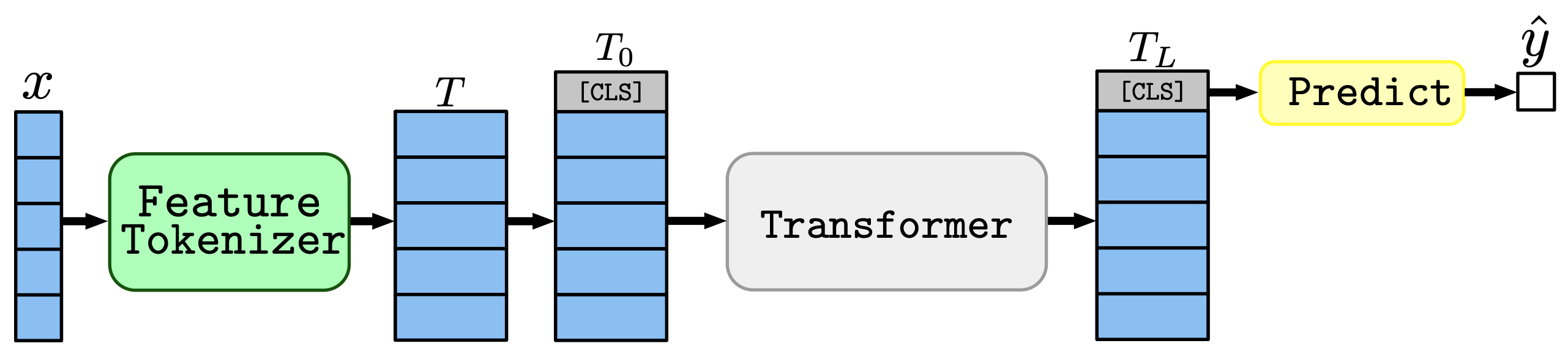The whole process is as follows: first, the input $x$ is converted into an embedding $T$ by the Feature Tokenizer, and then $T0$, to which a [CLS] token is added, is passed through the Transformer. Then, the prediction is made based on the representation corresponding to the last [CLS] token.

The Feature Tokenizer module converts the input $x$ into an embedding $T \in R^{k×d}$.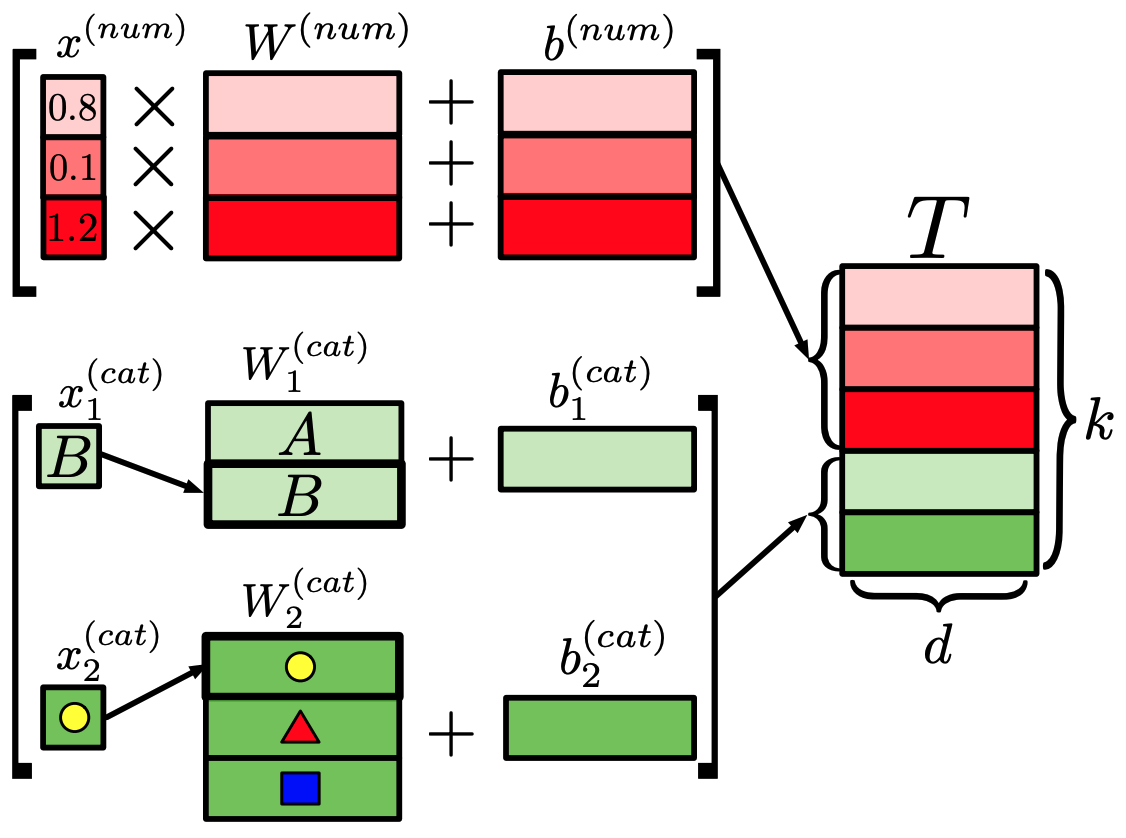At this time, depending on whether the input $x$ is numerical data ($x^{(num)}$) or categorical data ($x^{(cat)}$), different processing is performed as shown in the following equation.

$T^{(num)}_j = b^{(num)}_j + x^{(num)}_j \cdot W^{(num)}_j \in R^d$

$T^{(cat)}_j = b^{(cat)}_j + e^T_j W^{(cat)}_j in R^d$.

$T = stack [T^{(num)}_1, ... , T^{(num)}_{k^{(num)}} , T^{(cat)}_1 , ... , T^{(cat)}_{k^{(cat)}} ] \in R^{k×d}$.

where $k$ is the number of features and $e^T_j$ is the one-hot vector corresponding to the categorical feature.

The Transformer process consists of $L$ Transformer layers $(F_1,..., F_L)$ shown in the following figure. , F_L)$as shown in the following figure.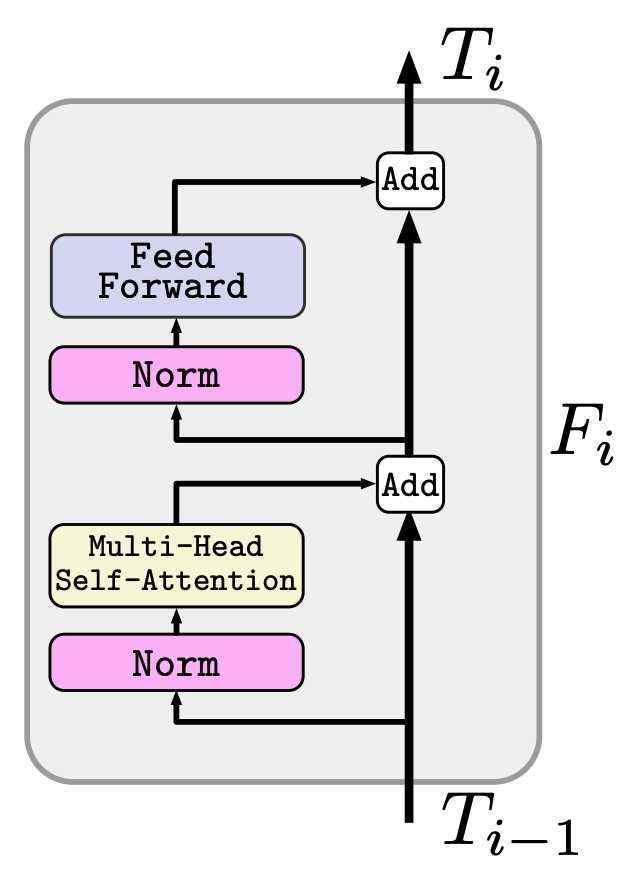About Prediction Using the [CLS] token representation obtained through Transformer, prediction is performed by the process represented by the following equation.$\hat{y} = Linear(ReLU(LayerNorm(T^{[CLS]}_L))))$As a caveat, FT-Transformer has some challenges compared to MLP and ResNet, such as the large resources required for training and the difficulty in applying it to a large number of features. (This could potentially be improved by using a more efficient Transformer variant.) Other models Among the existing models specialized for tabular data, those used in the comparison experiments are the following experimental results In this section, we compare the performance of different architectures. We do not employ model-independent methods such as pre-training or data augmentation. data set For our experiments, we use 11 different datasets, which are listed below.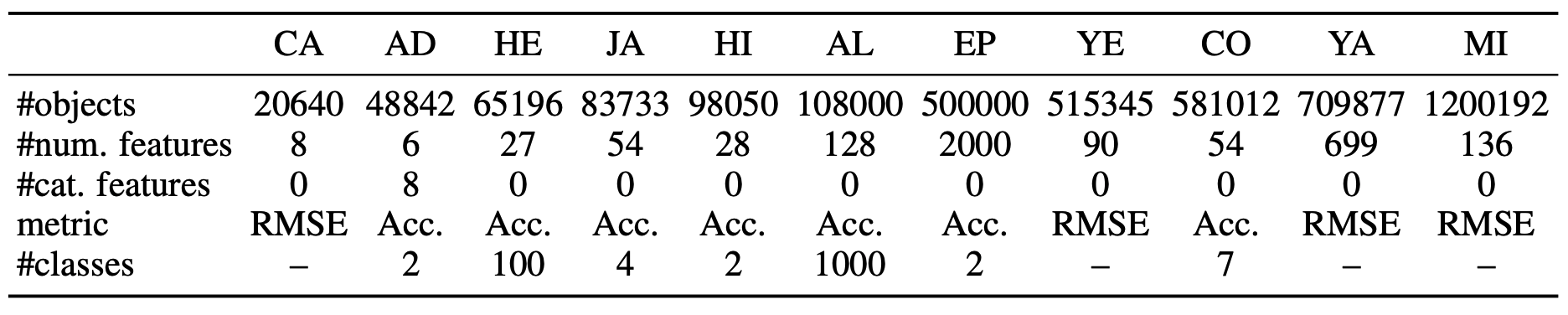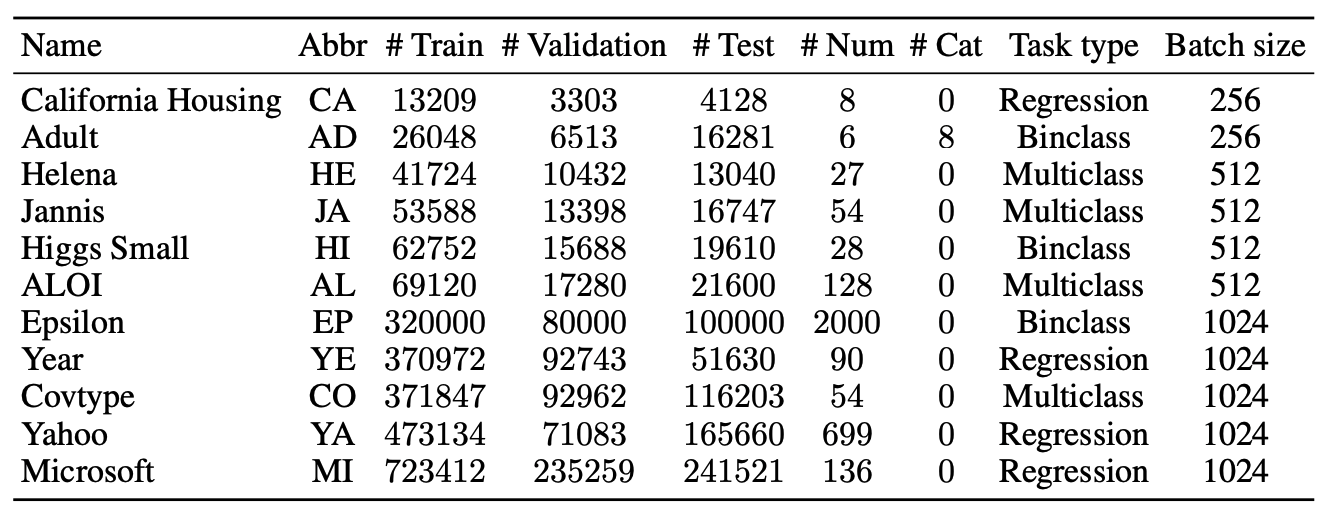(RMSE stands for Root-Mean-Square Error and Acc stands for Accuracy.) To evaluate the performance, we run 15 experiments with different random seeds. In the ensemble setting, we also split the 15 models into 5 groups of 5 and use the average of the predictions within each group (see the original paper for other settings). Comparison of deep learning models The experimental results of the deep learning model are as follows.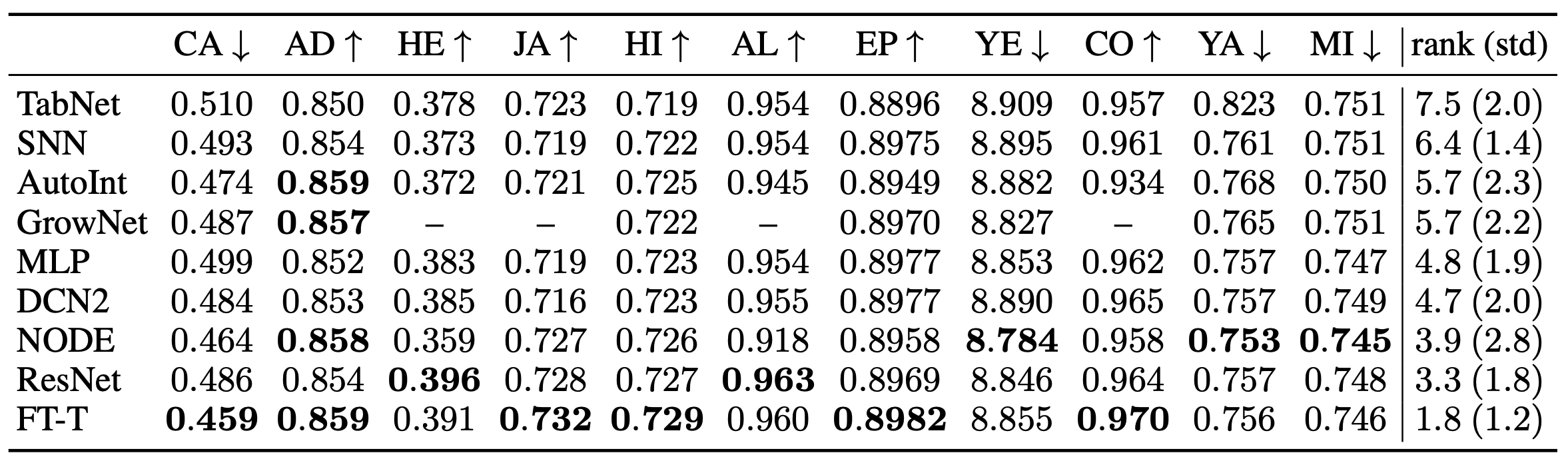The rightmost rank in the table shows the average rank of each model. Among the baselines introduced in the paper, FT-Transformer (FT-T) shows the best performance in most of the tasks and is a very powerful model. ResNet was also found to be an effective baseline, showing the second-best results after FT-Transformer despite its simplicity. Among the other methods, NODE shows the best results. The results in the ensemble setting are also shown below.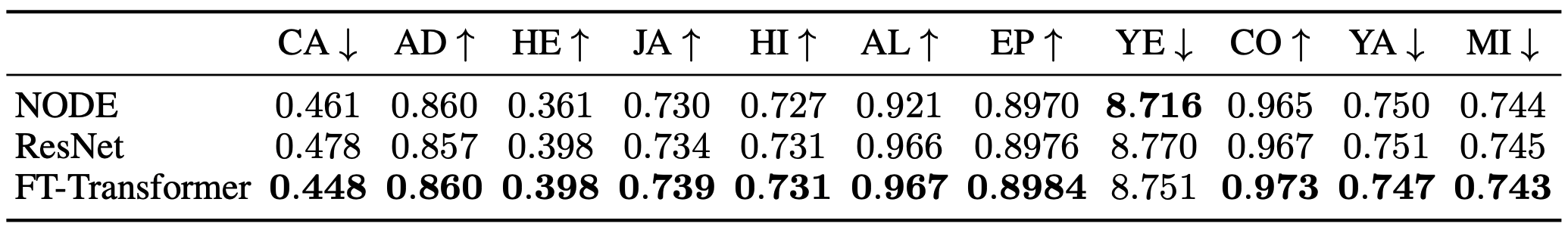In the ensemble setting, ResNet and FT-Transformer showed even better results. Comparison of deep learning models and GBDT Next, we compare deep learning models with GBDT. However, we ignore speed, hardware requirements, etc., and compare them with the best performance that each method can achieve. Since GBDT includes an ensemble technique, the deep learning model also uses the results of the ensemble setting for comparison. The results are as follows.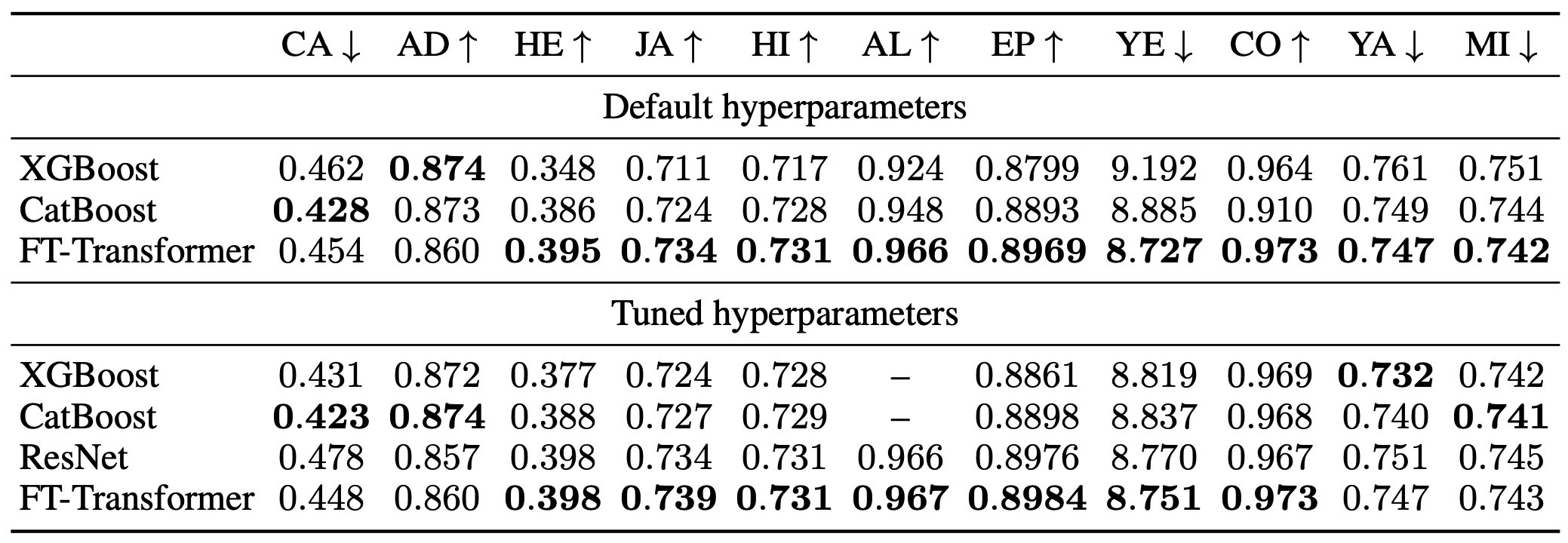Default hyperparameters show the default hyperparameter settings and Tuned hyperparameters show the results of the hyperparameter tuned model. The FT-Transformer performs equally well with both default and tuned instruments, indicating that it is possible to build an excellent ensemble model without tuning. We also found that GBDT performed better on some tasks in the tuned setting and that we cannot assume that deep learning models will always outperform GBDT. (The deep learning model seems to be superior in the comparison of the number of tasks only, but this may just be because the benchmark was biased towards problems suitable for the deep learning model.) However, FT-Transformer shows good results in all tasks, and it is a more universal model for tabular data than other methods. In general, we cannot say that there is always a method that is the best solution between DL models and GBDT. It can also be said that future research on deep learning methods should focus on datasets where GBDT outperforms deep learning methods. Comparison between FT-Transformer and ResNet To compare the universality of FT-Transformer and ResNet, we conduct experiments using a synthesis task. Specifically, for$f_{GBDT}$, which represents the average prediction of 30 randomly constructed decision trees, and$f_{DL}$, which is an MLP with three randomly initialized hidden layers, we create the following synthetic data.$x ~ N(0, I_k), y = \alpha \cdot f_{GBDT}(x) + (1 - \alpha) \cdot f_{DL}(x)$This synthetic task is considered to be more suitable for GBDT when$\alpha$is large, and more suitable for deep learning methods when$\alpha\$ is small.

The comparison of each method in this synthesis task is as follows.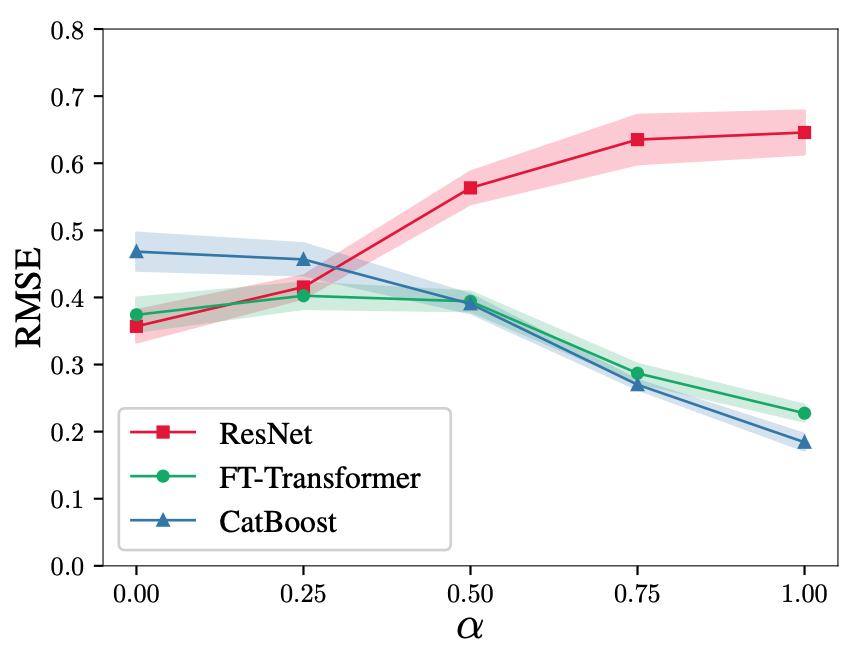As shown in the figure, the performance of ResNet deteriorates significantly in GBDT-oriented settings, while FT-Transformer shows generally good results, revealing the universality of FT-Transformer.

Finally, a comparison of the learning time between ResNet and FT-Transformer is shown below.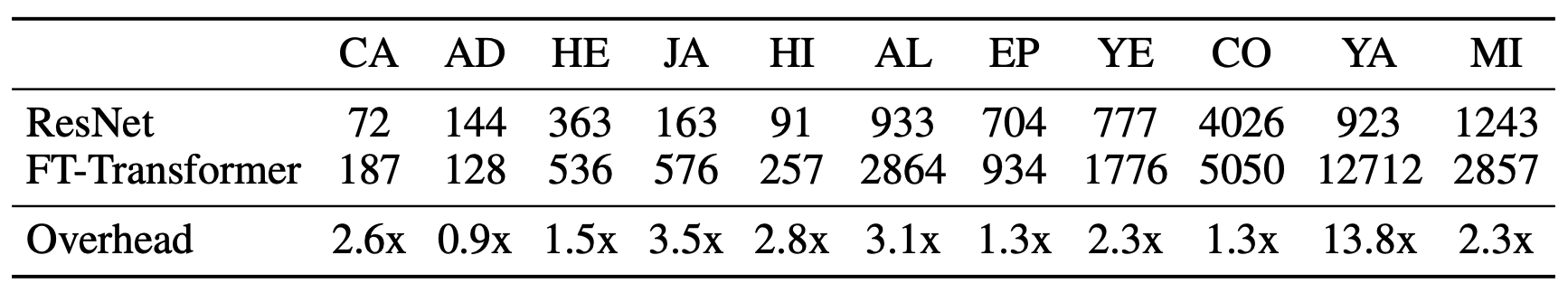In general, FT-Transformer requires more time for training, which is especially noticeable on datasets with a large number of features (YA); the large computational cost of FT-Transformer is an important issue to be improved in the future.

summary

In the paper presented in this article, we experimented with deep learning models on tabular data by introducing a simple baseline and comparing it with existing methods.

It includes various information on deep learning methods for tabular data, such as the proposed ResNet-based model, which is a useful baseline for deep learning methods on tabular data, the proposed FT-Transformer as an example of applying the Transformer to tabular data, and a comparison with current deep learning methods and GBDT. method on tabular data, comparison with current deep learning methods and GBDT, etc. It contains various information about the method and the official code is also available. If you are interested, please see the original paper as well.

If you have any suggestions for improvement of the content of the article,